In Part 11, let’s see how to create bar charts in R. Let’s create a simple bar chart using the `barplot()` command, which is easy to use. First, we set up a vector of numbers. Then we count them using the `table()` command, and then we plot them. The `table()` command creates a simple table of counts of the elements in a dataset.
`H <- c(2,3,3,3,4,5,5,5,5,6)`
Now we count the elements using the `table()` command, as follows:
```counts <- table(H) counts H```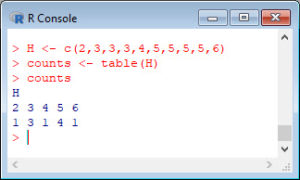Now we plot the counts.
`barplot(counts)`The horizontal axis records the values in your dataset, while the vertical axis gives the counts of each value. You will see that the `barplot(`) command does not perform the count directly, so we use the `table()` command first.
You can plot your data directly if we omit the `table()` command. Now, the height of the bars matches the values in the dataset. This is a useful technique if your data are already in the form of counts or if you wish to plot the magnitudes of each element.
```B <- c(3, 2, 25, 37, 22, 34, 19) barplot(B, col="darkgreen")```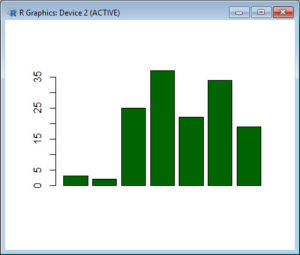Here we have one bar for each element, and the height gives the value of the element.
Now, let’s create a more complex barplot using various arguments, some of which you have already met in previous blogs.
`barplot(B, main="My new barplot", xlab="Letters", ylab="My Y values", names.arg=c("A","B","C","D","E","F","G"), border="red", density=c(90, 70, 50, 40, 30, 20, 10))`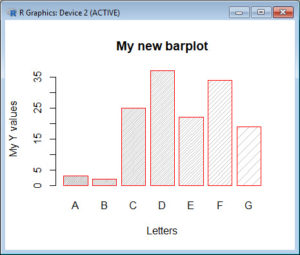You have seen the following arguments before: `main`, `xlab` and `ylab`, but not the others. OK. What did the `density` parameter achieve? Try other values of the `density` parameter and see what you get. What does the command `names.arg` do?
OK. Let’s create a third, more complex, bar chart, based on several (four) variables. Let’s assume that you wish to plot the magnitudes of each value within each variable.
```data <- structure(list(W= c(1L, 3L, 6L, 4L, 9L), X = c(2L, 5L, 4L, 5L, 12L), Y = c(4L, 4L, 6L, 6L, 16L), Z = c(3L, 5L, 6L, 7L, 6L)), .Names = c("W", "X", "Y", "Z"), class = "data.frame", row.names = c(NA, -5L)) attach(data) print(data)```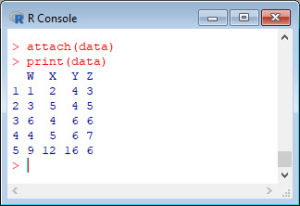Now we wish to create side-by-side bar charts for the four variables, `W`, `X`, `Y` and `Z`. First, we set up some colours, one for each of the five values within each variable.
`colours <- c("red", "orange", "blue", "yellow", "green")`
Now, plot a bar chart of the four variables, with adjacent bars, using the `as.matrix()` command and the argument `beside = T`.
`barplot(as.matrix(data), main="My Barchart", ylab = "Numbers", cex.lab = 1.5, cex.main = 1.4, beside=TRUE, col=colours)`What did the argument `beside = TRUE` achieve?
Now we create a legend at the top-left corner. To create a legend without a frame, include: `bty="n"`. The `bty` argument controls legend borders.
`legend("topleft", c("First","Second","Third","Fourth","Fifth"), cex=1.3, bty="n", fill=colours)`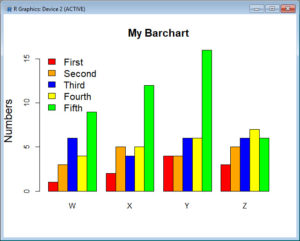We used the `topleft` argument to position the legend towards the top left of the chart. Other options include:
`"bottomright"`, `"bottom"`, `"bottomleft"`, `"left"`, `"topleft"`, `"top"`, `"topright"`, `"right"`, `"center"`.
Try these for yourselves.
That wasn’t so hard! In Blog 12 we will look at further plotting techniques in R.
See you later!
David

#### Annex: R codes used

```# Create a vector of numbers
H <- c(2,3,3,3,4,5,5,5,5,6)

# Create a simple table of counts of the elements in a dataset
counts <- table(H)
counts
H

# Create a bar chart
barplot(counts)

# Create a bar chart from a vector of counts without using table() command
B <- c(3, 2, 25, 37, 22, 34, 19)
barplot(B, col="darkgreen")

# Create a more complete bar chart
barplot(B, main="My new bar chart", xlab="Letters", ylab="My Y values", names.arg=c("A","B","C","D","E","F","G"), border="red", density=c(90, 70, 50, 40, 30, 20, 10))

# Generate a dataset
data <- structure(list(W= c(1L, 3L, 6L, 4L, 9L), X = c(2L, 5L, 4L, 5L, 12L), Y = c(4L, 4L, 6L, 6L, 16L), Z = c(3L, 5L, 6L, 7L, 6L)), .Names = c("W", "X", "Y", "Z"), class = "data.frame", row.names = c(NA, -5L))
attach(data)
print(data)

# Create a vector with colour names
colours <- c("red", "orange", "blue", "yellow", "green")

# Create a bar chart for a new dataset
barplot(as.matrix(data), main="My Barchart", ylab = "Numbers", cex.lab = 1.5, cex.main = 1.4, beside=TRUE, col=colours)

# Insert a legend to existing bar chart
legend("topleft", c("First","Second","Third","Fourth","Fifth"), cex=1.3, bty="n", fill=colours)
```